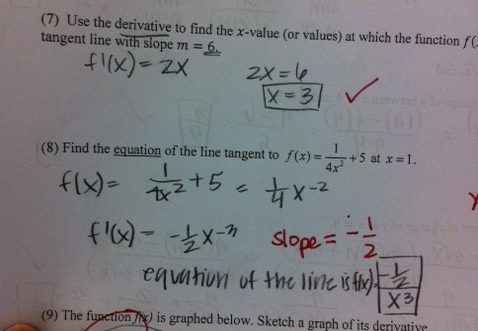Categories

# Lines Tangent to a PointWhat does the student know? What doesn’t the student know yet?

## 2 replies on “Lines Tangent to a Point”

If we assume this student shows understanding in the correct first solution (I am becoming increasingly sceptical of whether calculations show understanding) then he or she understands that slope has to do with the derivative, and that the information leads to a an equation to find x. But then perhaps the student does not understand tangent, or does but lacks understanding or mastery of finding equations of lines. Or just ran out of time? But seriously, why should we assume this student understands anything at all, instead of just having memorized some procedures?

I agree with Julia, but I also would say that it is likely that when the class discussed the relationship between the graphs of f and f’ (assessed by question 8 on the test above) that the student got things mixed up in their minds. To a student who is only partly listening or only partially present, it is very easy to not see the difference between the two types of problems (although they are clearly worlds apart in the mind of a calc teacher).